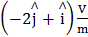# The magnetic field of an electromagnetic wave is given by= 1.6 × 10 −6 cos(2 × 107z + 6 × 1015t)The associated electric field will be (a)= 4.8 × 102 cos(2 × 107z + 6 × 1015t)(b)= 4.8 × 102 cos(2 × 107z  − 6 × 1015t)(c)= 4.8 × 102 cos(2 × 107z  − 6 ×1015t)(d)= 4.8 × 102 cos(2 × 107z + 6  × 1015t)## Question ID - 1 :- The magnetic field of an electromagnetic wave is given by= 1.6 × 10 −6 cos(2 × 107z + 6 × 1015t)The associated electric field will be (a)= 4.8 × 102 cos(2 × 107z + 6 × 1015t)(b)= 4.8 × 102 cos(2 × 107z  − 6 × 1015t)(c)= 4.8 × 102 cos(2 × 107z  − 6 ×1015t)(d)= 4.8 × 102 cos(2 × 107z + 6  × 1015t)3537

Amplitude of electric field, E = B0C

= 1.6 × 10 −6 ×× 3 × 108

= 4.8 × 102V/m

Also×is along(the direction of propagation)

⇒= 4.8 × 102 cos(2 × 107z + 6 × 1015t)Next Question :
 In the circuit shown, a four −wire potentiometer is made of a 400 cm long wire, which extends between A and B. The resistance per unit length of the potentiometer wire is r = 0.01/ cm. If an ideal voltmeter is connected as shown with jockey J at 50 cm from end A, the expected reading of the voltmeter will be(a) 0.75 V (b) 0.50 V (c) 0.20 V (d) 0.25 V